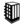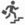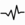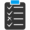Algorithm - Interview Questions and Answers for 'Sorting algorithm' | Search Interview Question - javasearch.buggybread.com# Search Interview QuestionsMore than 3000 questions in repository.There are more than 900 unanswered questions.Have a video suggestion.
Click Correct / Improve and please let us know.
Label / Company      Label / Company / TextSubmit Question

Algorithm - Interview Questions and Answers for 'Sorting algorithm' - 6 question(s) found - Order By NewestQ1. Explain various Searching and Sorting Algorithms ? AlgorithmThis question was recently asked at 'HeadStrong,ServiceNow'.This question is still unanswered. Can you please provide an answer.Help us improve. Please let us know the company, where you were asked this question :LikeDiscussCorrect / ImproveSearch Algorithm  Sorting AlgorithmAsked in 2 CompaniesbasicfrequentQ2. Which sorting algorithm is used by Collections.sort() in Java ? Core Java
Ans. The sorting algorithm is a modified mergesort. This algorithm offers guaranteed n log(n) performance.Help us improve. Please let us know the company, where you were asked this question :LikeDiscussCorrect / Improvejava   algorithm   collections   collections.sort   sorting algorithmexpertTry 1 Question(s) TestQ3. Write any sorting algorithm. AlgorithmHelp us improve. Please let us know the company, where you were asked this question :LikeDiscussCorrect / ImproveAlgorithm  Sorting AlgorithmAsked in 4 CompaniesbasicfrequentQ4. Explain In place sorting algorithm ? AlgorithmThis question was recently asked at 'Reddit,ServiceNow'.This question is still unanswered. Can you please provide an answer.Help us improve. Please let us know the company, where you were asked this question :LikeDiscussCorrect / Improvesorting algorithm   in place sorting algorithmAsked in 2 CompaniesQ5. Write a Program for selection sort.
Ans. public class SelectionSort {
public static void main(String[] args){
int a[] = {1,3,4,5,7,8,2,6};

for(int i=0;i<a.length;i++){
for(int j=0;j<i;j++){
if(a[j] > a[j]){
int temporary = a[j];
a[j] = a[i];
a[i] = temporary;
}
}
}

for(int i=0;i<a.length;i++){
System.out.println(a[i]);
}
}
}Help us improve. Please let us know the company, where you were asked this question :LikeDiscussCorrect / Improvesorting algorithm  selection sortQ6. Write a program for bubble sort.
Ans. public class BubbleSort {
public static void main(String[] args){
int a[] = {1,3,4,5,7,8,2,6};

for(int i=0;i<a.length-1;i++){
for(int j=i;j<a.length;j++){
if(a[j] < a[j]){
int temporary = a[j];
a[j] = a[i];
a[i] = temporary;
}
}
}

for(int i=0;i<a.length;i++){
System.out.println(a[i]);
}
}
}Help us improve. Please let us know the company, where you were asked this question :LikeDiscussCorrect / Improvesorting algorithm  Bubble sort

## Help us and Others Improve. Please let us know the questions asked in any of your previous interview.

Any input from you will be highly appreciated and It will unlock the application for 10 more requests.